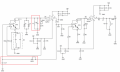# How to satisfy the oscillating condition in my circuit.

#### Ngô Đình Nhân

Joined Jan 8, 2017
45
Hi everyone
I am going to design a oscillator circuit with Quartz Crystal and BJT now. However, I actually do not understand about the " phase shift " in this circuit. Please see my circuit at bottom pictureThis is a oscillator circuit, (OTA just is a transconductance transitor , ~ " ideal transitor"), and two conditions for oscillator circuit is: A.B =1, and total phase shift is 2k*pi ( k = 0,1,2,...). And my problem related to " totally phase shift ". In this circuit, as far as I know, the signal at pin (B) and pin (C) of OTA is out of phase while the maximum phase shift of low pass filter ( C17 + RW3) is 90 degree. So " how does the circuit oscillate ? ".

And I think that the tank circuit ( L1+C8 ) will be induce a 90 degree of shift phase. Am I right ?

Moreover, when I design this circuit with a certain quartz crystal ( fundamental mode is F0 ), I will follow by these steps:
Step 1. I will calculate the value of C17 & RW3 firstly. Choose any value of C17 and value of RW3 will be calculate by value of C17 and F0 and required phase shift 180 degree. From this, I will know about Gain of Feedback
Step 2: Select the value of R11 & R5 &RW1 to satisfy the condition A.B =1
Step 3: For value of L1 & C8, I will choose any value of L1, and then calculate C8.